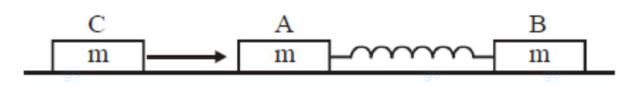# Two identical blocks A and B each of mass m

Question:

Two identical blocks $A$ and $B$ each of mass $m$

resting on the smooth horizontal floor are connected by a light spring of natural length $L$ and spring constant $\mathrm{K}$. A third block $\mathrm{C}$ of mass $\mathrm{m}$ moving with a speed $\mathrm{v}$ along the line joining $\mathrm{A}$ and $\mathrm{B}$ collides with $\mathrm{A}$. The maximum compression in the spring is1. (1) $\sqrt{\frac{\mathrm{M}}{2 \mathrm{~K}}}$

2. (2) $\sqrt{\frac{\mathrm{mv}}{2 \mathrm{~K}}}$

3. (3) $\sqrt{\frac{\mathrm{mv}}{\mathrm{K}}}$

4. (4) $\sqrt{\frac{\mathrm{m}}{2 \mathrm{~K}}}$

Correct Option: 1

Solution:

(1)

$\mathrm{C}$ comes to rest

$V_{c m}$ of $A \& B=\frac{v}{2}$

$\Rightarrow \frac{1}{2}$ is $\mathrm{v}_{\mathrm{ret}}^{2}=\frac{1}{2} \mathrm{kx}^{2}$\

$x=\sqrt{\frac{\mu \times v^{2}}{k}}=\sqrt{\frac{m}{2 k}} v$